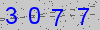# Let’s get started! What should not appear at the beginning of a sentence

It is important to begin your sentences correctly. You may be wondering what is meant by this. The easiest way to answer this question is to examine what should not appear at the beginning of your sentence.

Numerals: You should never initiate a sentence with a numeral. If you see a numeral at the beginning, know that it is always incorrect. There are several ways to resolve this issue. Let’s look at an example and a few possible solutions to correct the problem. Incorrect sentence: “23 rats were randomly divided into several groups.”

Solution #1: “There were 23 rats that were randomly divided into several groups.” The writer can simply eliminate the issue by prefacing the number with “There were.”

Solution #2: “Twenty-three rats were randomly divided into several groups.” While numbers 1–9 are typically spelled out and numbers 10 and above are represented by numerals in many style guides, spelling out the number at the beginning of the sentence (no matter how small or large) is an acceptable way to convey the information and be grammatically correct.

And: You should never start a sentence with “and.” Let’s look at another example. Incorrect sentence: “And 23 rats were randomly divided into several groups.”

Solution #1: To solve this problem, the writer can simply remove “and” from the beginning and then use one of the solutions mentioned above so that the numeral is not a problem.

Solution #2: The writer can also preface the sentence with “In addition” so it becomes: “In addition, 23 rats were randomly divided into several groups.”

Solution #3: Depending upon the sentence that precedes the one in question, the writer can also simply combine it with the previous sentence. Let’s say the first sentence is: “The rats were allowed to acclimate for two weeks.” The writer can put the two sentences together: “The rats were allowed to acclimate for two weeks before they were randomly divided into several groups.”

What’s at the beginning of your sentence?

Security Code*